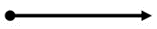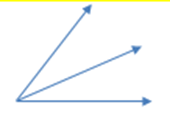# Access Point #: MAFS.4.MD.3.AP.7a (Archived Access Point)

This document was generated on CPALMS - www.cpalms.org

Find sums of angles that show a ray (adjacent angles).

#### Essential Understandings

Concrete:

• Identify a ray (a ray is a line that has a start but no end).• Identify adjacent angles (angles that have a common side and a common vertex).Representation:
• Understand the following concepts, symbols and vocabulary: adjacent, ray, vertex, side, angle, sum, addition, degree, °.

 Number: MAFS.4.MD.3.AP.7a Category: Access Points Cluster: Geometric measurement: understand concepts of angle and measure angles. (Additional Cluster) :  Clusters should not be sorted from Major to Supporting and then taught in that order. To do so would strip the coherence of the mathematical ideas and miss the opportunity to enhance the major work of the grade with the supporting clusters.

#### Related Standards

 Name Description MAFS.4.MD.3.7: Recognize angle measure as additive. When an angle is decomposed into non-overlapping parts, the angle measure of the whole is the sum of the angle measures of the parts. Solve addition and subtraction problems to find unknown angles on a diagram in real world and mathematical problems, e.g., by using an equation with a symbol for the unknown angle measure.

#### Related Courses

 Name Description 5012060: Mathematics - Grade Four 7712050: Access Mathematics Grade 4 5020110: STEM Lab Grade 4 5012055: Grade 3 Accelerated Mathematics 5012015: Foundational Skills in Mathematics 3-5

#### Element Cards

 Name Description 4th Grade Math Element Cards: Element Cards are available to assist in planning for instruction. They are designed to promote understanding of how students move toward the academic standards. Element Cards contain one or more access points, essential understandings, suggested instructional strategies and suggested supports.

#### Teaching Idea

 Name Description Curriculum Resource Guide: Measurement and Geometry: Complete Unit